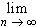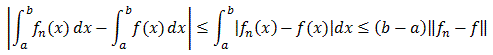#Interactive Real Analysis

Next | Previous | Glossary | Map

## 8.2. Uniform Convergence

### Theorem 8.2.7: Uniform Convergence and Integration

Let fn(x) be a sequence of continuous functions defined on the interval [a, b] and assume that fn converges uniformly to a function f. Then f is Riemann-integrable andfn(x) dx =fn(x) dx =f(x) dx

Since fn are continuous and converge uniformly to f, the limit function must be continuous. In particular all functions must therefore be Riemann integrable. Also:Since the right side goes to zero as n goes to infinity we are done.Next | Previous | Glossary | Map